# Mathaid geometry

Highly interactive tutorials and self-test system for individual e-learning, home schooling, college computer learning centers, and distance learning. The product emphasizes on building problem-solving skills. Tutorials include the reviews of basic concepts, interactive examples, and standard problems with randomly generated parameters. The self-test system allows selecting topics and length for a test, saving test results, and getting the test review. Topics covered: parallel lines, angles of a convex polygon, congruent triangles, quadrilaterals, similarity, right triangles, trigonometry, circles (arcs and angles, lengths of segments), constructions, areas of plane figures, solids, coordinate geometry, transformations (translations, reflections, rotations, dilations). The demo version contains selected lessons from the full version. The demo is designed to demonstrate the functionality and all features of the product.

## Related software (5)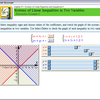## MathAid Algebra II

Tutorial contains basic concepts, interactive examples, and problems with randomly generated parameters. A customer is allowed to select chapters for a test, get the test review, ...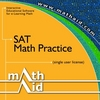## MathAid SAT. Math Practice

Tutorial contains basic concepts, interactive examples, and problems with randomly generated parameters. A customer is allowed to select chapters for a test, get the test review, ...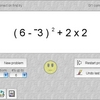## Smooth Operators

Smooth Operators is a complete solution for learning, practicing, and testing the order of operations. An interactive lesson teaches concepts. Practice by clicking operands, or tes ...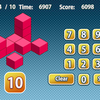## Count the Cubes

Can you spot the exact number of cubes immediately?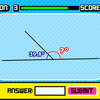## Geometry Quiz

Challenge your mind and try to solve all equations.